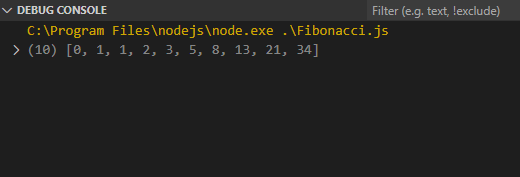GFG App
Open AppBrowser
Continue

# How to calculate the Fibonacci series in JavaScript ?

Fibonacci series is a number series that contains integers in the following pattern.

`0, 1, 1, 2, 3, 5, 8, 13, 21, 34, 55, ..`

In terms of mathematics, the general formula for calculating the Fibonacci series is

`fn = fn-1 + fn-2 , where n ≥ 2`

Here, f0 = 0 and f1 = 1.

We need to calculate n Fibonacci numbers for any given integer n, where n≥0.

Example:

```Input   : n = 5
Output  : [0, 1, 1, 2, 3]

Input   : n = 10
Output  : [0, 1, 1, 2, 3, 5, 8, 13, 21, 34]```

In this article, we are focusing on two major and common ways for calculating the Fibonacci series.

1. Using For loop and While loop
2. Using recursion

Using Loop: The method of calculating Fibonacci Series using this method is better as compared to the recursive method. This method makes use of Dynamic Programming which works by storing the number generated so far and then using it for further calculations.

As the number for n=1 and n=2 are fixed, i.e, 0 and 1, then the rest of the numbers in the series can be calculated by the logic,

```f3 = f2 + f1
f4 = f3 + f2
f5 = f4 + f3
...
fn = fn-1 + fn-2```

This logic can be implemented using for as well as the while loop in JavaScript.

Using for loop: As the first two values of the series are fixed, we start the loop with i = 2 and iterate until i < n, because array indexing starts at 0, so, n = 1 will technically mean i = 0 in case of arrays.

## HTML

 `<``script``> ` `    ``const n = 10; ` `     `  `    ``// Create a new array of size 'n' ` `    ``var series = new Array(n);  ` ` `  `    ``// Fills all places in array with 0 ` `    ``series.fill(0);    ` `     `  `    ``// Seed value for 1st element ` `    ``series = 0;  ` `     `  `    ``// Seed value for 2nd element ` `    ``series = 1;   ` `     `  `    ``for(let i = 2; i < ``n``; i++) { ` ` `  `        ``// Apply basic Fibonacci formulae ` `        ``series[i] = series[i-1] + series[i-2];   ` `    ``} ` ` `  `    ``// Print the series ` `    ``console.log(series); ` ``

Output:Fibonacci series up till 10th element gets printed

Using while loop:

## HTML

 `<``script``> ` `    ``const n = 10; ` `     `  `    ``// Create a new array of size 'n' ` `    ``var series = new Array(n);  ` `     `  `    ``// Fills all places in array with 0 ` `    ``series.fill(0);   ` `     `  `    ``// Seed value for 1st element ` `    ``series = 0;  ` `     `  `    ``// Seed value for 2nd element ` `    ``series = 1;   ` `     `  `    ``// Initialize the conditional variable ` `    ``let i = 2;   ` `    ``while(i < ``n``) { ` ` `  `        ``// Apply basic Fibonacci formulae ` `        ``series[i] = series[i-1] + series[i-2];   ` `        `  `        ``// Increment the conditional variable ` `        ``i++;   ` `    ``} ` `     `  `    ``// Print the series ` `    ``console.log(series);  ` ``

Output:Fibonacci series up till 10th element gets printed

Using Recursion: The recursion method to print the whole Fibonacci series till a certain number is not recommended because, recursion algorithm itself is costly in terms of time and complexity, and along with fetching a Fibonacci series number at a certain position, we need to store them in an array, which calls the recursive function again and again for each and every element, i.e, n times!

The recursion method can be applied as follows in JavaScript.

## HTML

 `<``script``> ` `    ``function fibonacci(n){ ` `      ``if(n == 1) return 0;  // Return value for n=1 ` `      ``if(n == 2) return 1;  // Return value for n=2 ` `     `  `      ``// Recursive call ` `      ``return fibonacci(n-1) + fibonacci(n-2);  ` `    ``} ` `     `  `    ``const n = 10; ` `     `  `    ``// Create a new array of size 'n' ` `    ``var series = new Array(n);  ` ` `  `    ``// Fills all places in array with 0 ` `    ``series.fill(0);    ` `     `  `    ``for(let i = 1; i <= n; i++) { ` ` `  `        ``// Store i-th Fibonacci number ` `        ``series[i-1] = fibonacci(i);  ` `    ``} ` ` `  `    ``// Print the series ` `    ``console.log(series); ` ``

Output:Fibonacci series up till 10th element gets printed

My Personal Notes arrow_drop_up